## Round Robin Scheduling-

In Round Robin Scheduling,

• CPU is assigned to the process on the basis of FCFS for a fixed amount of time.
• This fixed amount of time is called as time quantum or time slice.
• After the time quantum expires, the running process is preempted and sent to the ready queue.
• Then, the processor is assigned to the next arrived process.
• It is always preemptive in nature.

 Round Robin Scheduling is FCFS Scheduling with preemptive mode.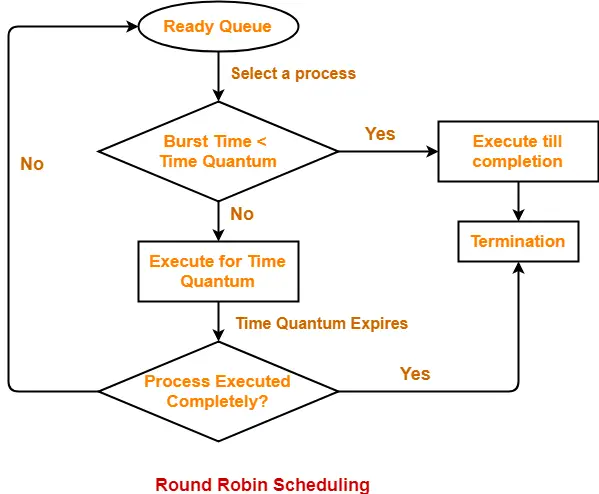• It gives the best performance in terms of average response time.
• It is best suited for time sharing system, client server architecture and interactive system.

• It leads to starvation for processes with larger burst time as they have to repeat the cycle many times.
• Its performance heavily depends on time quantum.
• Priorities can not be set for the processes.

## Important Notes-

### Note-01:

With decreasing value of time quantum,

• Number of context switch increases
• Response time decreases
• Chances of starvation decreases

Thus, smaller value of time quantum is better in terms of response time.

### Note-02:

With increasing value of time quantum,

• Number of context switch decreases
• Response time increases
• Chances of starvation increases

Thus, higher value of time quantum is better in terms of number of context switch.

### Note-03:

• With increasing value of time quantum, Round Robin Scheduling tends to become FCFS Scheduling.
• When time quantum tends to infinity, Round Robin Scheduling becomes FCFS Scheduling.

### Note-04:

• The performance of Round Robin scheduling heavily depends on the value of time quantum.
• The value of time quantum should be such that it is neither too big nor too small.

## Problem-01:

Consider the set of 5 processes whose arrival time and burst time are given below-

 Process Id Arrival time Burst time P1 0 5 P2 1 3 P3 2 1 P4 3 2 P5 4 3

If the CPU scheduling policy is Round Robin with time quantum = 2 unit, calculate the average waiting time and average turn around time.

## Solution-

### Gantt Chart-

P5, P1, P2, P5, P4, P1, P3, P2, P1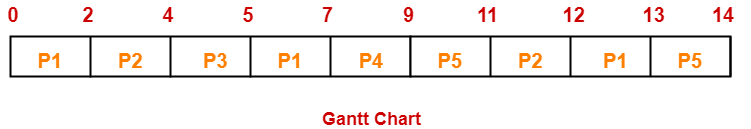Now, we know-

• Turn Around time = Exit time – Arrival time
• Waiting time = Turn Around time – Burst time

Also read- Various Times of Process

 Process Id Exit time Turn Around time Waiting time P1 13 13 – 0 = 13 13 – 5 = 8 P2 12 12 – 1 = 11 11 – 3 = 8 P3 5 5 – 2 = 3 3 – 1 = 2 P4 9 9 – 3 = 6 6 – 2 = 4 P5 14 14 – 4 = 10 10 – 3 = 7

Now,

• Average Turn Around time = (13 + 11 + 3 + 6 + 10) / 5 = 43 / 5 = 8.6 unit
• Average waiting time = (8 + 8 + 2 + 4 + 7) / 5 = 29 / 5 = 5.8 unit

## Problem-02:

Consider the set of 6 processes whose arrival time and burst time are given below-

 Process Id Arrival time Burst time P1 0 4 P2 1 5 P3 2 2 P4 3 1 P5 4 6 P6 6 3

If the CPU scheduling policy is Round Robin with time quantum = 2, calculate the average waiting time and average turn around time.

## Solution-

### Gantt chart-

P5, P6, P2, P5, P6, P2, P5, P4, P1, P3, P2, P1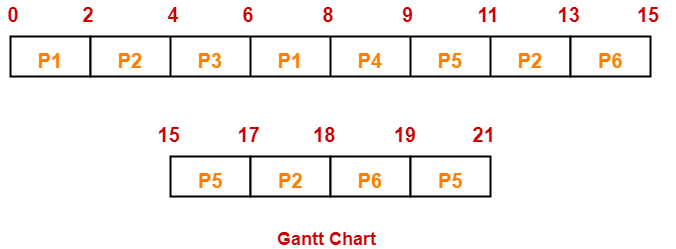Now, we know-

• Turn Around time = Exit time – Arrival time
• Waiting time = Turn Around time – Burst time

 Process Id Exit time Turn Around time Waiting time P1 8 8 – 0 = 8 8 – 4 = 4 P2 18 18 – 1 = 17 17 – 5 = 12 P3 6 6 – 2 = 4 4 – 2 = 2 P4 9 9 – 3 = 6 6 – 1 = 5 P5 21 21 – 4 = 17 17 – 6 = 11 P6 19 19 – 6 = 13 13 – 3 = 10

Now,

• Average Turn Around time = (8 + 17 + 4 + 6 + 17 + 13) / 6 = 65 / 6 = 10.84 unit
• Average waiting time = (4 + 12 + 2 + 5 + 11 + 10) / 6 = 44 / 6 = 7.33 unit

## Problem-03:

Consider the set of 6 processes whose arrival time and burst time are given below-

 Process Id Arrival time Burst time P1 5 5 P2 4 6 P3 3 7 P4 1 9 P5 2 2 P6 6 3

If the CPU scheduling policy is Round Robin with time quantum = 3, calculate the average waiting time and average turn around time.

## Solution-

### Gantt chart-

P3, P1, P4, P2, P3, P6, P1, P4, P2, P3, P5, P4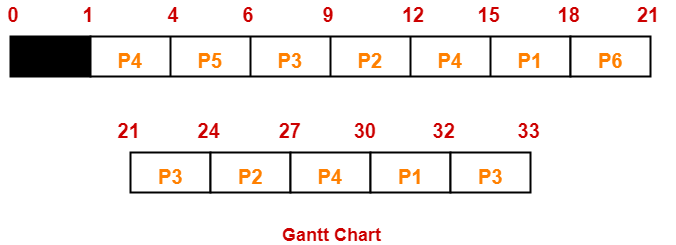Now, we know-

• Turn Around time = Exit time – Arrival time
• Waiting time = Turn Around time – Burst time

 Process Id Exit time Turn Around time Waiting time P1 32 32 – 5 = 27 27 – 5 = 22 P2 27 27 – 4 = 23 23 – 6 = 17 P3 33 33 – 3 = 30 30 – 7 = 23 P4 30 30 – 1 = 29 29 – 9 = 20 P5 6 6 – 2 = 4 4 – 2 = 2 P6 21 21 – 6 = 15 15 – 3 = 12

Now,

• Average Turn Around time = (27 + 23 + 30 + 29 + 4 + 15) / 6 = 128 / 6 = 21.33 unit
• Average waiting time = (22 + 17 + 23 + 20 + 2 + 12) / 6 = 96 / 6 = 16 unit

## Problem-04:

Four jobs to be executed on a single processor system arrive at time 0 in the order A, B, C, D. Their burst CPU time requirements are 4, 1, 8, 1 time units respectively. The completion time of A under round robin scheduling with time slice of one time unit is-

1. 10
2. 4
3. 8
4. 9

## Solution-

 Process Id Arrival time Burst time A 0 4 B 0 1 C 0 8 D 0 1

### Gantt chart-

C, A, C, A, C, A, D, C, B, A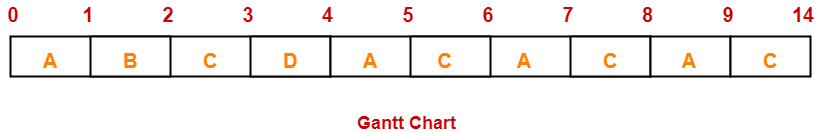Clearly, completion time of process A = 9 unit.

Thus, Option (D) is correct.

To gain better understanding about Round Robin Scheduling,

Watch this Video Lecture

Next Article- Priority Scheduling

Get more notes and other study material of Operating System.

Watch video lectures by visiting our YouTube channel LearnVidFun.

## SJF Scheduling-

In SJF Scheduling,

• Out of all the available processes, CPU is assigned to the process having smallest burst time.
• In case of a tie, it is broken by FCFS Scheduling.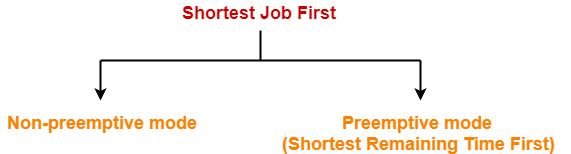• SJF Scheduling can be used in both preemptive and non-preemptive mode.
• Preemptive mode of Shortest Job First is called as Shortest Remaining Time First (SRTF).

• SRTF is optimal and guarantees the minimum average waiting time.
• It provides a standard for other algorithms since no other algorithm performs better than it.

• It can not be implemented practically since burst time of the processes can not be known in advance.
• It leads to starvation for processes with larger burst time.
• Priorities can not be set for the processes.
• Processes with larger burst time have poor response time.

## Problem-01:

Consider the set of 5 processes whose arrival time and burst time are given below-

 Process Id Arrival time Burst time P1 3 1 P2 1 4 P3 4 2 P4 0 6 P5 2 3

If the CPU scheduling policy is SJF non-preemptive, calculate the average waiting time and average turn around time.

## Solution-

### Gantt Chart-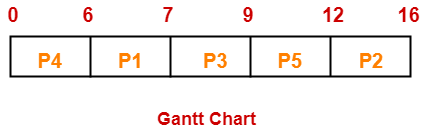Now, we know-

• Turn Around time = Exit time – Arrival time
• Waiting time = Turn Around time – Burst time

Also read- Various Times of Process

 Process Id Exit time Turn Around time Waiting time P1 7 7 – 3 = 4 4 – 1 = 3 P2 16 16 – 1 = 15 15 – 4 = 11 P3 9 9 – 4 = 5 5 – 2 = 3 P4 6 6 – 0 = 6 6 – 6 = 0 P5 12 12 – 2 = 10 10 – 3 = 7

Now,

• Average Turn Around time = (4 + 15 + 5 + 6 + 10) / 5 = 40 / 5 = 8 unit
• Average waiting time = (3 + 11 + 3 + 0 + 7) / 5 = 24 / 5 = 4.8 unit

## Problem-02:

Consider the set of 5 processes whose arrival time and burst time are given below-

 Process Id Arrival time Burst time P1 3 1 P2 1 4 P3 4 2 P4 0 6 P5 2 3

If the CPU scheduling policy is SJF preemptive, calculate the average waiting time and average turn around time.

## Solution-

### Gantt Chart-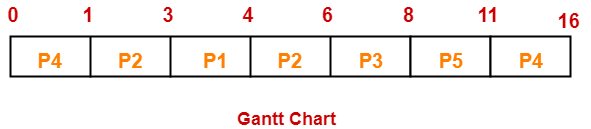Now, we know-

• Turn Around time = Exit time – Arrival time
• Waiting time = Turn Around time – Burst time

 Process Id Exit time Turn Around time Waiting time P1 4 4 – 3 = 1 1 – 1 = 0 P2 6 6 – 1 = 5 5 – 4 = 1 P3 8 8 – 4 = 4 4 – 2 = 2 P4 16 16 – 0 = 16 16 – 6 = 10 P5 11 11 – 2 = 9 9 – 3 = 6

Now,

• Average Turn Around time = (1 + 5 + 4 + 16 + 9) / 5 = 35 / 5 = 7 unit
• Average waiting time = (0 + 1 + 2 + 10 + 6) / 5 = 19 / 5 = 3.8 unit

## Problem-03:

Consider the set of 6 processes whose arrival time and burst time are given below-

 Process Id Arrival time Burst time P1 0 7 P2 1 5 P3 2 3 P4 3 1 P5 4 2 P6 5 1

If the CPU scheduling policy is shortest remaining time first, calculate the average waiting time and average turn around time.

## Solution-

### Gantt Chart-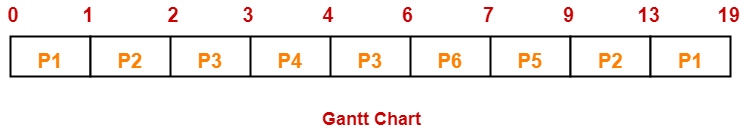Now, we know-

• Turn Around time = Exit time – Arrival time
• Waiting time = Turn Around time – Burst time

 Process Id Exit time Turn Around time Waiting time P1 19 19 – 0 = 19 19 – 7 = 12 P2 13 13 – 1 = 12 12 – 5 = 7 P3 6 6 – 2 = 4 4 – 3 = 1 P4 4 4 – 3 = 1 1 – 1 = 0 P5 9 9 – 4 = 5 5 – 2 = 3 P6 7 7 – 5 = 2 2 – 1 = 1

Now,

• Average Turn Around time = (19 + 12 + 4 + 1 + 5 + 2) / 6 = 43 / 6 = 7.17 unit
• Average waiting time = (12 + 7 + 1 + 0 + 3 + 1) / 6 = 24 / 6 = 4 unit

## Problem-04:

Consider the set of 3 processes whose arrival time and burst time are given below-

 Process Id Arrival time Burst time P1 0 9 P2 1 4 P3 2 9

If the CPU scheduling policy is SRTF, calculate the average waiting time and average turn around time.

## Solution-

### Gantt Chart-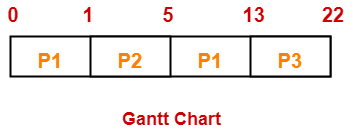Now, we know-

• Turn Around time = Exit time – Arrival time
• Waiting time = Turn Around time – Burst time

 Process Id Exit time Turn Around time Waiting time P1 13 13 – 0 = 13 13 – 9 = 4 P2 5 5 – 1 = 4 4 – 4 = 0 P3 22 22- 2 = 20 20 – 9 = 11

Now,

• Average Turn Around time = (13 + 4 + 20) / 3 = 37 / 3 = 12.33 unit
• Average waiting time = (4 + 0 + 11) / 3 = 15 / 3 = 5 unit

## Problem-05:

Consider the set of 4 processes whose arrival time and burst time are given below-

 Process Id Arrival time Burst time P1 0 20 P2 15 25 P3 30 10 P4 45 15

If the CPU scheduling policy is SRTF, calculate the waiting time of process P2.

## Solution-

### Gantt Chart-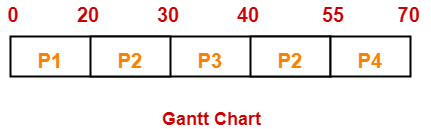Now, we know-

• Turn Around time = Exit time – Arrival time
• Waiting time = Turn Around time – Burst time

Thus,

• Turn Around Time of process P2 = 55 – 15 = 40 unit
• Waiting time of process P2 = 40 – 25 = 15 unit

## Implementation of Algorithm-

• Practically, the algorithm can not be implemented but theoretically it can be implemented.
• Among all the available processes, the process with smallest burst time has to be selected.
• Min heap is a suitable data structure where root element contains the process with least burst time.
• In min heap, each process will be added and deleted exactly once.
• Adding an element takes log(n) time and deleting an element takes log(n) time.
• Thus, for n processes, time complexity = n x 2log(n) = nlog(n)

To gain better understanding about SJF Scheduling,

Watch this Video Lecture

Next Article- Techniques to Predict Burst Time

Get more notes and other study material of Operating System.

Watch video lectures by visiting our YouTube channel LearnVidFun.

## FCFS Scheduling-

In FCFS Scheduling,

• The process which arrives first in the ready queue is firstly assigned the CPU.
• In case of a tie, process with smaller process id is executed first.
• It is always non-preemptive in nature.

• It is simple and easy to understand.
• It can be easily implemented using queue data structure.
• It does not lead to starvation.

• It does not consider the priority or burst time of the processes.
• It suffers from convoy effect.

### Convoy Effect

In convoy effect,

• Consider processes with higher burst time arrived before the processes with smaller burst time.
• Then, smaller processes have to wait for a long time for longer processes to release the CPU.

## Problem-01:

Consider the set of 5 processes whose arrival time and burst time are given below-

 Process Id Arrival time Burst time P1 3 4 P2 5 3 P3 0 2 P4 5 1 P5 4 3

If the CPU scheduling policy is FCFS, calculate the average waiting time and average turn around time.

## Solution-

### Gantt Chart-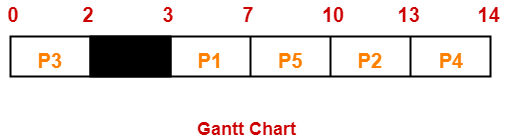Here, black box represents the idle time of CPU.

Now, we know-

• Turn Around time = Exit time – Arrival time
• Waiting time = Turn Around time – Burst time

Also read- Various Times of Process

 Process Id Exit time Turn Around time Waiting time P1 7 7 – 3 = 4 4 – 4 = 0 P2 13 13 – 5 = 8 8 – 3 = 5 P3 2 2 – 0 = 2 2 – 2 = 0 P4 14 14 – 5 = 9 9 – 1 = 8 P5 10 10 – 4 = 6 6 – 3 = 3

Now,

• Average Turn Around time = (4 + 8 + 2 + 9 + 6) / 5 = 29 / 5 = 5.8 unit
• Average waiting time = (0 + 5 + 0 + 8 + 3) / 5 = 16 / 5 = 3.2 unit

## Problem-02:

Consider the set of 3 processes whose arrival time and burst time are given below-

 Process Id Arrival time Burst time P1 0 2 P2 3 1 P3 5 6

If the CPU scheduling policy is FCFS, calculate the average waiting time and average turn around time.

## Solution-

### Gantt Chart-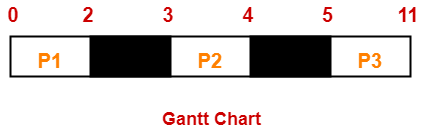Here, black box represents the idle time of CPU.

Now, we know-

• Turn Around time = Exit time – Arrival time
• Waiting time = Turn Around time – Burst time

 Process Id Exit time Turn Around time Waiting time P1 2 2 – 0 = 2 2 – 2 = 0 P2 4 4 – 3 = 1 1 – 1 = 0 P3 11 11- 5 = 6 6 – 6 = 0

Now,

• Average Turn Around time = (2 + 1 + 6) / 3 = 9 / 3 = 3 unit
• Average waiting time = (0 + 0 + 0) / 3 = 0 / 3 = 0 unit

## Problem-03:

Consider the set of 6 processes whose arrival time and burst time are given below-

 Process Id Arrival time Burst time P1 0 3 P2 1 2 P3 2 1 P4 3 4 P5 4 5 P6 5 2

If the CPU scheduling policy is FCFS and there is 1 unit of overhead in scheduling the processes, find the efficiency of the algorithm.

## Solution-

### Gantt Chart-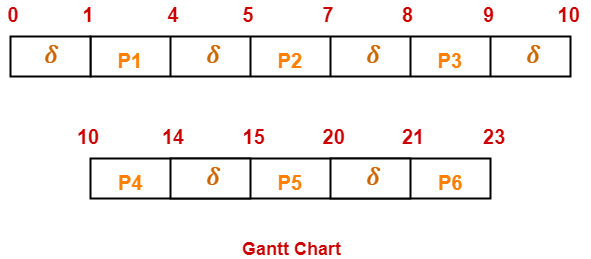Here, δ denotes the context switching overhead.

Now,

• Useless time / Wasted time = 6 x δ = 6 x 1 = 6 unit
• Total time = 23 unit
• Useful time = 23 unit – 6 unit = 17 unit

Efficiency (η)

= Useful time  / Total Total

= 17 unit / 23 unit

= 0.7391

= 73.91%

To gain better understanding about FCFS Scheduling,

Watch this Video

Next Article- SJF Scheduling | SRTF Scheduling

Get more notes and other study material of Operating System.

Watch video lectures by visiting our YouTube channel LearnVidFun.

## Various Times Related To Process-

Before you go through this article, make sure that you have gone through the previous article on Process States.

Various times related to process are-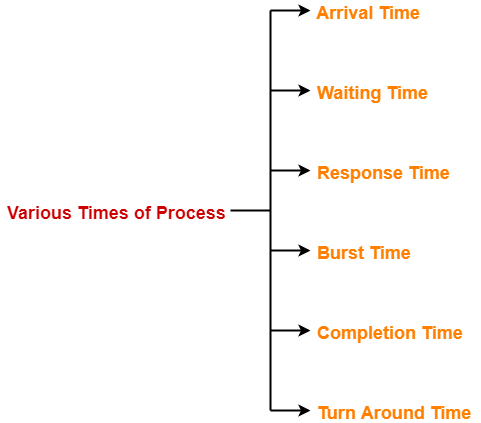1. Arrival time
2. Waiting time
3. Response time
4. Burst time
5. Completion time
6. Turn Around Time

## 1. Arrival Time-

• Arrival time is the point of time at which a process enters the ready queue.

## 2. Waiting Time-

• Waiting time is the amount of time spent by a process waiting in the ready queue for getting the CPU.

 Waiting time = Turn Around time – Burst time

## 3. Response Time-

• Response time is the amount of time after which a process gets the CPU for the first time after entering the ready queue.

 Response Time = Time at which process first gets the CPU – Arrival time

## 4. Burst Time-

• Burst time is the amount of time required by a process for executing on CPU.
• It is also called as execution time or running time.
• Burst time of a process can not be known in advance before executing the process.
• It can be known only after the process has executed.

## 5. Completion Time-

• Completion time is the point of time at which a process completes its execution on the CPU and takes exit from the system.
• It is also called as exit time.

## 6. Turn Around Time-

• Turn Around time is the total amount of time spent by a process in the system.
• When present in the system, a process is either waiting in the ready queue for getting the CPU or it is executing on the CPU.

 Turn Around time = Burst time + Waiting time

OR

 Turn Around time = Completion time – Arrival time

## Important Note-

While discussing the above definitions,

• We have considered that the process does not require an I/O operation.
• When process is present in the system, it will be either waiting for the CPU in the ready state or it will be executing on the CPU.

To gain better understanding about various times of process,

Watch this Video Lecture

Next Article- FCFS Algorithm | CPU Scheduling

Get more notes and other study material of Operating System.

Watch video lectures by visiting our YouTube channel LearnVidFun.